Question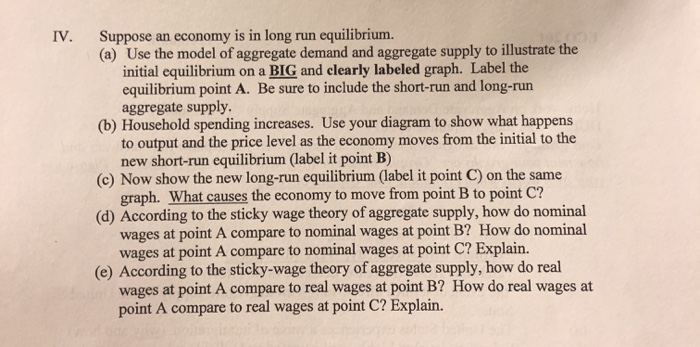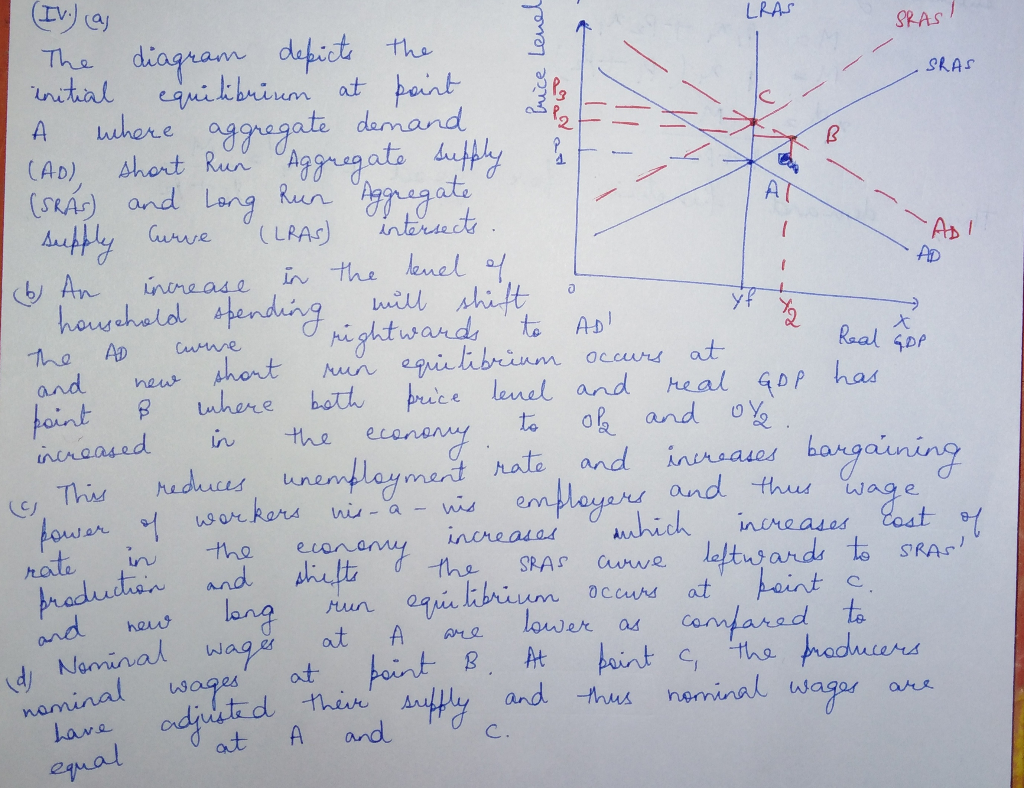#### Earn Coins

Coins can be redeemed for fabulous gifts.

Similar Homework Help Questions
• ### QUESTION 5-1 Chapter 14 Suppose economy is in long run equilibrium. [Only one diagram is required...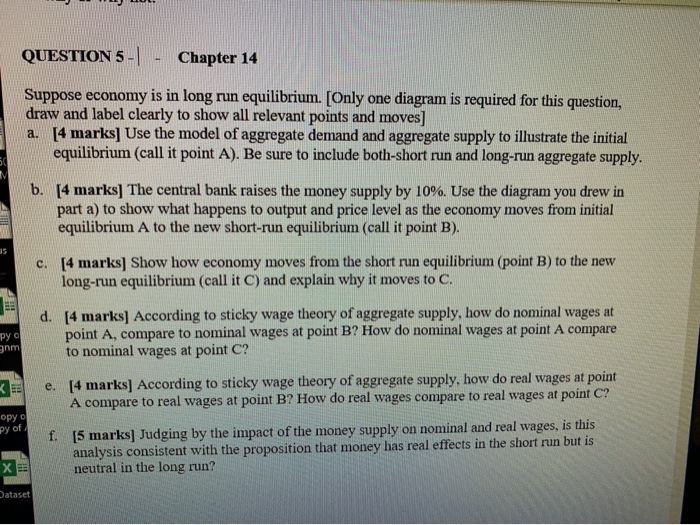QUESTION 5-1 Chapter 14 Suppose economy is in long run equilibrium. [Only one diagram is required for this question, draw and label clearly to show all relevant points and moves] a. [4 marks] Use the model of aggregate demand and aggregate supply to illustrate the initial equilibrium (call it point A). Be sure to include both-short run and long-run aggregate supply. b. [4 marks] The central bank raises the money supply by 10%. Use the diagram you drew in part...

• ### The graph shows the economy in long-run equilibrium Then the world economy expands and the demand...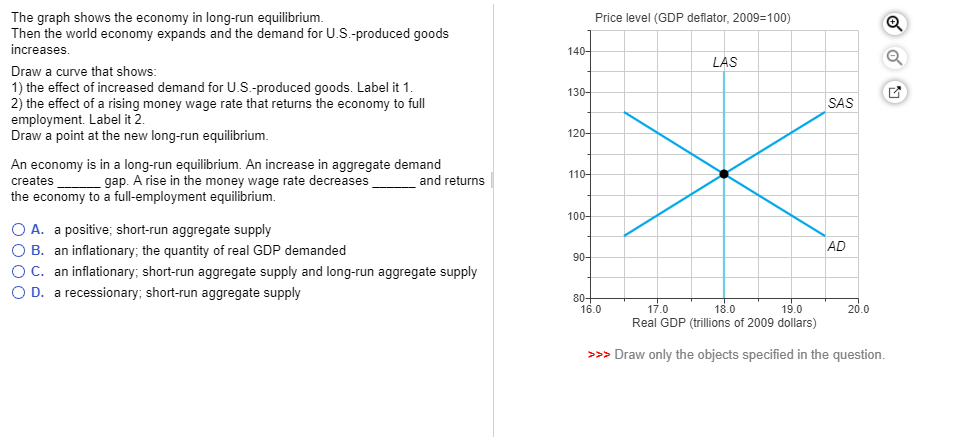The graph shows the economy in long-run equilibrium Then the world economy expands and the demand for U.S.-produced goods increases Price level (GDP deflator, 2009-100) 14 Draw a curve that shows 1) the effect of increased demand for U.S.-produced goods. Label it 1 2) the effect of a rising money wage rate that returns the economy to full employment. Label it 2. Draw a point at the new long-run equilibrium 13 SAS 12 An economy is in a long-run equilibrium....

• ### If the economy begins at long-run equilibrium at potential output when a negative aggregate demand shock...

If the economy begins at long-run equilibrium at potential output when a negative aggregate demand shock occurs,l initially there will be a ____________________ equilibrium because prices are _____________ in the short run. a. new long-run equilibrium below potential output, sticky b. new short-run equilibrium below potential output, sticky c. new short-run equilibrium above potential output, sticky d. new short-run equilibrium below potential output, flexible

• ### The figure below depicts the aggregate demand curve (AD), the short-run aggregate supply curve (SRAS), and the long-run aggregate supply curve (LRAS) for the United States. The economy is initially at long-run equilibrium, at point A.The figure below depicts the aggregate demand curve (AD), the short-run aggregate supply curve (SRAS), and the long-run aggregate supply curve (LRAS) for the United States. The economy is initially at long-run equilibrium, at point A.One of the most contentious issues among economists involves the economy’s adjustment to long-run equilibrium. Some economists believe that adjustment can and should occur naturally. This group, the classical economists, stress the importance of aggregate supply. Others see the return to long-run equilibrium as an...

• ### Using aggregate demand (AD), short-run aggregate supply (SRAS) and long-run aggregate supply (LRAS) curves

Question 1: AD-SRAS-LRAS Model Using aggregate demand (AD), short-run aggregate supply (SRAS) and long-run aggregate supply (LRAS) curves, graphically illustrate the effect of an increase in the money supply on output and prices in the short and long run. Assume that the economy is initially in long run equilibrium at the potential output level and prices are fixed in the short-run. In your graph, label "A" for the initial equilibrium, "B' for the short-run equilibrium, and "C" for the long-run equilibrium.

• ### The Queensland economy is initially in long-run equilibrium. But the economy is hit by a price...

The Queensland economy is initially in long-run equilibrium. But the economy is hit by a price increase in imported fertilizers which are essential for the state's agricultural sector. In the short run, the short-run aggregate supply curve shifts left. In the long run, the price level is lower than its original value, output returns to potential, and real wages increase. In the short run, the short-run aggregate supply curve shifts right. In the long run, the price level returns to...

• ### Number 2. If the price level increases to 107 then youur s Question Completion Status The economy enters the long-run once: O Nominal wages become real wages o Real wages become nominal wages suff...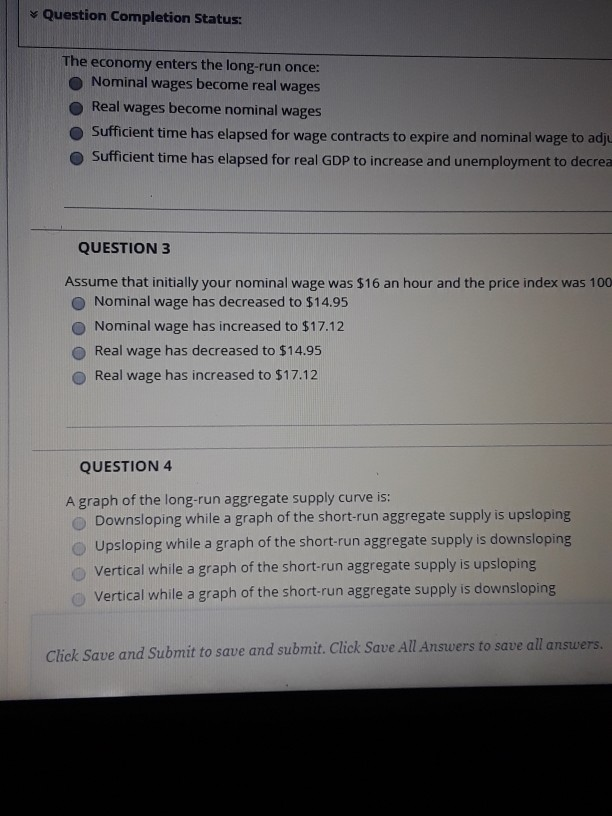Number 2. If the price level increases to 107 then youur s Question Completion Status The economy enters the long-run once: O Nominal wages become real wages o Real wages become nominal wages sufficient time has elapsed for wage contracts to expire and nominal wage to adj O Sufficient time has elapsed for real GDP to increase and unemployment to decrea QUESTION 3 Assume that initially your nominal wage was \$16 an hour and the price index was 100 O...

• ### 6) Suppose an economy is in its long-run equilibrium and the real money demand function for...

6) Suppose an economy is in its long-run equilibrium and the real money demand function for the economy is (M/P) d =0.2Y. Now suppose suddenly money demand becomes (M/P) d =0.4Y. a) Draw a graph and show the initial long-run equilibrium and then show the short-run equilibrium that would results from this change in money demand [Hint: You are drawing the graph from Chapter 10 but think about how this money demand change affects velocity from Chapter 5.] b) Assuming...

• ### If the economy begins at long-run equilibrium at potential output when a negative aggregate demand shock occurs, l initi...

If the economy begins at long-run equilibrium at potential output when a negative aggregate demand shock occurs, l initially there will be a ____________________ equilibrium because prices are _____________ in the short run. a. new long-run equilibrium below potential output, sticky b.new short-run equilibrium below potential output, sticky c.new short-run equilibrium above potential output, sticky d.new short-run equilibrium below potential output, flexible

• ### Aggregate supply and aggregate demand in Lithuania were in their long run equilibrium. Then consumers decided...

Aggregate supply and aggregate demand in Lithuania were in their long run equilibrium. Then consumers decided to spend less and save more. In a well-labeled graph, show how aggregate demand, aggregate supply, and the equilibrium change in both the short and long run Explain what happened to the economy, especially the price level and output, in the short and long run . Show (in a pair of graphs) what the central bank could do to offset the decrease in consumer...